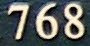Friday, October 28, 2011

768

768 = 28 x 3.

768 has a unique representation as a sum of three squares: 768 = 162 + 162 + 162.

768 is a divisor of 654 - 1.

768 is the number of subsets of {1, 2, 3, . . . , 12} that have an integer average.

416,768 = 7682 - 4162.RSA-768 was a member of set of large semiprimes (RSA Numbers) that were part of the RSA Factoring Challenge.